Economic Dispatch for Power System included Wind and Solar Thermal energy

Saoussen BRINI, Hsan Hadj ABDALLAH, and Abderrazak OUALI

ENIS, Dép. Génie Electrique, 3038 Sfax, Tel.:74 274 088

E-mails: ingenieurbrini@yahoo.fr abderrazak.ouali@enis.rnu.tn

Abstract

With the fast development of technologies of alternative energy, the electric power network can be composed of several renewable energy resources. The energy resources have various characteristics in terms of operational costs and reliability. In this study, the problem is the Economic Environmental Dispatching (EED) of hybrid power system including wind and solar thermal energies. Renewable energy resources depend on the data of the climate such as the wind speed for wind energy, solar radiation and the temperature for solar thermal energy. In this article it proposes a methodology to solve this problem. The resolution takes account of the fuel costs and reducing of the emissions of the polluting gases. The resolution is done by the Strength Pareto Evolutionary Algorithm (SPEA) method and the simulations have been made on an IEEE network test (30 nodes, 8 machines and 41 lines).

Keywords

economic dispatch, total cost, active losses, multi objectives optimization, evolutionary algorithms, SPEA, renewable energy

Introduction

The economic and environmental problems in the power generation have received considerable attention. The apparition of the energy crisis and the excessive increase of the consumption have obliged production companies to implant renewable sources. However, this production poses many technical problems for their integration in the electric system.

The economic dispatch [8, 16] is a significant function in the modern energy system. It consists in programming correctly the electric production in order to reduce the operational cost [4, 7, 10, 15]. Recently, the wind power and solar thermal power attracted much attention like promising renewable energy resources [1, 6, 11, 18, 19, 22].

The problem is formulated as a multiobjective optimization problem [3, 5, 9, 24, 25]. It consists in distributing the active and renewable productions between the power stations of the most economic way, to reduce the emissions of the polluting gases and to maintain the stability of the network after penetration of renewable energy. The number of decision variables of the problem is related to all the nodes of the network.

Renewable energy

In this study, it is interested in two types of energies; wind power and thermal solar energy.

Wind energy

The mechanical power recovered by a wind turbine can be written in the form [6, 17, 23]: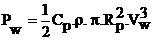(1)

where CP, is the aerodynamic coefficient of turbine power (it characterizes the aptitude of the aerogenerator to collect wind power), ρ is the air density, Rp the turbine ray and  VW wind speed. The power coefficient value CP, depends on the rotation speed of turbine and wind speed.

Mechanical adjustment of the wind power

Wind turbine is dimensioned to develop a nominal power Pn from a nominal wind speed Vn. For wind speeds higher than Vn, the wind mill must modify these aerodynamic parameters in order to avoid the mechanical overloads, so that the power recovered by the turbine does not exceed the nominal power for which the wind mill was designed.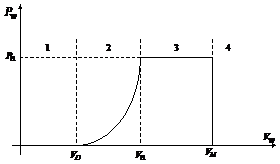Figure 1. Diagram of the useful power according to the wind speed.

According to the figure1, the characteristic of power according to the wind speed comprises four zones. Zone 1, where Pw = 0, zone 2, in which useful power depends on wind speed. Vw, zone 3, generally where provided power Pw remains appreciably equal to Pn and finally zone 4,Pw = 0

Solar energy

Solar energy is energy produced by the solar radiation, directly or in a diffuse way through the atmosphere. Thanks to various processes, it can be transformed into another form of useful energy for the human activity, in particular in electricity or heat [14, 17, 23].

The maximum power provided by a solar panel is given by the following characteristic [14, 17]: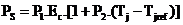(2)

Ec is solar radiation, Tjref is the reference temperature of the panels of 25°C, Tj is the cells junction temperature (°C), P1 represent the characteristic dispersion of the panels and the value for one panel is included enters 0.095 to 0.105 and the parameter P2=-0.47%/C°; is the drift in panels temperature .

The addition of one parameter P3 to the characteristic, gives more satisfactory results: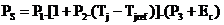(3)

This simplified model makes it possible to determine the maximum power provided by a group of panels for solar radiation and panel temperature given, with only three constant parameters P1, P2 and P3 and simple equation to apply.

A thermal solar power station consists of a production of solar system of heat which feeds from the turbines in a thermal cycle of electricity production.

Formulation of problem

The control system problem can be treated as follows:

Of absence of the auxiliary elements, the problem consists in extracting the maximum of power from the renewable sources. Then, we slice this power of the total demand PD. the remaining total demand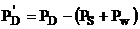, will distributed between the thermal power stations. The problem is reduced for a speed wind Vw and solar radiation Ec given to minimize the thermal cost functions and the emissions of polluting gases.

To approach to the reality, it is obvious that to must take account of the variation of the wind and solar radiation that can be done by using the techniques of the neurons networks which consists in forming a data base for various wind speed Vw, solar radiation Ec and total power demand PD. The neurons network is composed of three layers, the entries layer is formed by Vw, Ec et PD; the hidden internal layer which the number of neurons is variable and the exit layer which consists of 10 neurons which represent the minimal cost F1, the emissions of polluting gases F2, the generating nodes powers . The structure of this network is given by the figure (2).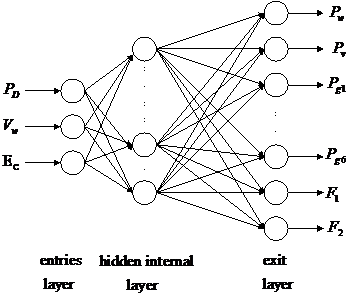Figure 2. Structure du réseau de neurones utilisé

Objective functions

Fuel cost function

The fuel cost function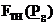in \$/h is represented by a quadratic function as follow [2, 5, 19]: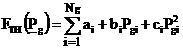(4)

The coefficients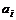,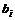and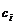are appropriate to every production unit,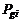is the real power output of i-th generator and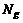is the number of thermal generators.

Emission fonction

The atmospheric emission can be represented by a function that links emissions with the power generated by every unit. The emission of SO2 depends on fuel consumption and has the same form as the fuel cost [8, 13].

The emission of NOx is difficult to predict and his production is associated to many factors as the temperature of the boiler and content of the air .

The emission function in ton/h which represents SO2 and NOx emission is a function of generator output and is expressed as follow :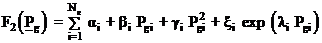(5)

Where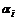,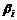and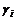are the coefficients of emission function corresponding to the i-th generator. These three parameters are determined by adjustment techniques of curves based on reel tests .

Problem constraints

The problem constraints are five types:

·        Production capacity constraints

The generated real power of each generator at the bus i is restricted by lower limit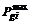and upper limit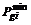: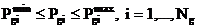(6)

·        Power balance constraint

The total power generation and the wind power must cover the total demand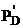and the power loss p in transmission lines, so we have: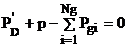(7)

·        Active power loss constraint

Active power loss of the transmission and transport lines, are positives: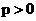(8)

·        Renewable power constraint:

The renewable power used for dispatch should not exceed the 30% of total power demand: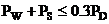(9)

Thus, the problem to be solved is formulated as follow:

·        Minimize:(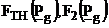)

Under: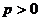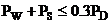Multi objectives Optimization

Principle

The multi-objective optimization problem is formulated in general as follow: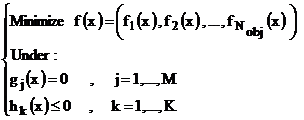(10)

with: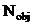: number of objectives functions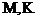: number of equality and inequality respectively constraints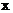: decision vector.

Two solutions x1 and x2 of such optimization problem, we could have one which dominates the other or none dominates the other.

In a minimization problem, a solution x1 dominates other solution x2 if the following two conditions are satisfied: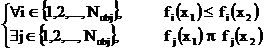(11)

Define bythe satisfiesability set, that is to say: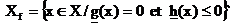where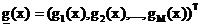and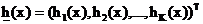A decision vector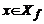is none dominated compared to a set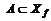, if: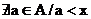(12)

The optimize solutions set that are non-dominated within the entire search space are denoted as Pareto-optimal and the set of objectives vectors corresponding constitute the Pareto-optimal set or Pareto-optimal front.

SPEA approach (Strength Pareto Evolutionary Algorithm)

In , Zitzler and Thiele propose an elitist evolutionary approach to solve a multi objective problem which is called Strength Pareto Evolutionary Algorithm (SPEA). The Elitism is introduced by an external Pareto set. This set stores the non-dominated solutions funded during the resolution of the problem. In order to reduce the size of the external set, an average linkage based on hierarchical clustering algorithm is used without destroying the characteristics of the trade-off front.

Noting by:

P : the current population.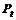: the external population.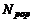: the size of current population.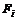the fitness of an individual i.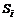the strength of an individual i.

The assignment procedure to calculate the fitness values is the following:

·        Step 1: For each individual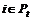is assigned a reel value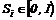called strength.is proportional to the number of individuals in the current population dominated by the individual i in the external Pareto set. It can be calculated as follows:

For an individual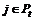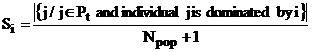(13)

The strength of a Pareto solution is also its fitness: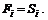·        Step 2: The fitness of an individualis the sum of the strengths of all external Pareto individualsdominated by. We add one in odder to guarantee that Pareto solutions are most likely to be produced.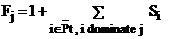(14)

where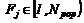The clustering algorithm is described by the following steps:

·        Step 1: To initialise clustering set C; each individualconstitutes a distinct cluster:(15)

·        Step 2: if the number of cluster is lower or equal to maximum size of external set (), go to step 5. Else, go to step 3.

·        Step 3: Calculate the distance between each pair of clusters. The distance dc between two clusters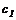and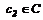is defined as the average distance between two pairs of individuals from each cluster: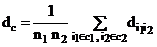(16)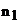and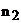are respectively the numbers of individuals in clusters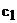and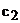.

·        Step 4: Find the pair of clusters corresponding to the minimal distance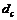between them. Combine into a large one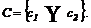and return to step 2.

·        Step 5: Find the centroid of each cluster. Select the nearest individual in this cluster to the centroid as a representative individual and remove all other individuals from the cluster.

·        Step 6: Thus, the reduced Pareto set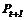is computed by uniting these representatives: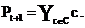Presentation of the test network

The structure of the test system is shown in fig.1.Appendix A1. It was derived from the standard IEEE 30-bus 6-generator test system while adding to him two renewable generators. The characteristics of the wind mill are presented in table 1. The values of the fuel and emission coefficients are given in table2. The lines data and bus data are given respectively in tables 1 and 2 in Appendix A1.

Table 1. Wind mill Data

 Characteristics of the wind mill propeller Diameter blades number Surface  swept chechmate Height Nominal wind speed Vn 34 m 3 1480 m2 45 m 15 m/s Nominal characteristics of the asynchronous generator Interlinked voltage Current Frequency power Pn Cos φ 660 V 760 A 50 Hz 790 Kw 0.91 Rs Rr Lσ Xm Rm 0.00374 Ω 0.00324 Ω 0.23 mH 5.8 mH 83.85 Ω

Table 2: Generator cost and emission coefficients

 G1 G2 G3 G4 G5 G6 Cost a 10 10 20 10 20 10 b 200 150 180 100 180 150 c 100 120 40 60 40 100 Emission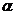4.091 2.543 4.258 5.326 4.258 6.131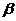-5.554 -6.047 -5.094 -3.550 -5.094 -5.555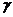6.490 5.638 4.586 3.380 4.586 5.151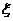2.0 10-4 5.0 10-4 1.0 10-6 2.0 10-3 1.0 10-6 1.0 10-5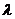2.857 3.333 8 2 8 6.667

Lower limit and upper limit of the generated real power of each generator at the bus it is shown by (17):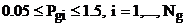(17)

Implementation and test of the neurons network

The neurons network is used to calculate in real time the active production in the thermal generating nodes and of the renewable origins. The structure of this network is given by the figure (2).

To ensure a good training of the neurons network, the base data is formed by 600 random solutions calculated by method SPEA and corresponding at a wind speed included enters 8 and 12 ms-1, solar radiation vary between 0w/m2 and 1000w/m2 and the total power demand vary between 0.8 and 4 pu.

Training curves of wind speed, solar radiation and the total power demand are given by figures (3, 4 and 5).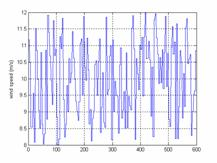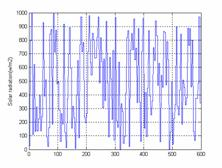Figure 3. Training curve of wind speed Figure 4. Training curve of solar radiation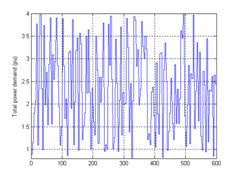Figure 5. Training curve of total power demand

During this phase, some of new examples are presented to the neurons network. The same examples were already simulated by SPEA method and we studied the quality of these answers given to table 3.

Table 3. Results of test neurons network

 Test exemple Pw (pu) Ps (pu) Pg1 (pu) Pg2 (pu) Pg3 (pu) Pg4 (pu) Pg5 (pu) Pg6 (pu) Emiss (ton/h) Coût (\$/h) PD1 2.35 0.6156 0.0475 0.3242 0.4352 0.4363 0.2052 0.3871 0.3795 0.1934 499.5495 PD2 3.43 0.7059 0.0175 0.4095 0.5220 0.5516 0.3635 0.5071 0.4846 0.1866 643.0993 PD3 3.35 0.8423 0.0385 0.3708 0.4870 0.5054 0.3575 0.4714 0.4605 0.1874 598.0519 PD4 1.16 0.3039 0.0252 0.3051 0.4284 0.4116 0.0939 0.3786 0.3598 0.1973 468.1725 PD5 0.93 0.2315 0.0412 0.3204 0.4743 0.4537 0.1231 0.3774 0.3504 0.1950 500.7442 PD6 1.25 0.3392 0.0301 0.3107 0.4249 0.4091 0.1017 0.3630 0.3410 0.1977 461.6945 PD7 2.65 0.4445 0.0266 0.3786 0.4834 0.5146 0.2245 0.4519 0.4197 0.1901 569.3929 PD8 3.10 0.5576 0.0388 0.4071 0.5251 0.5541 0.3173 0.5067 0.4879 0.1871 638.1037 Response of neurons network Pw (pu) Ps (pu) Pg1 (pu) Pg2 (pu) Pg3 (pu) Pg4 (pu) Pg5 (pu) Pg6 (pu) Emiss (ton/h) Coût     (\$/h) PD1 2.35 0.6156 0.0475 0.3202 0.4351 0.4317 0.2163 0.3874 0.3767 0.1932 499.6342 PD2 3.43 0.7059 0.0175 0.4105 0.5289 0.5448 0.3633 0.5111 0.4931 0.1864 643.0030 PD3 3.35 0.8423 0.0385 0.3711 0.4872 0.5114 0.3614 0.4711 0.4611 0.1872 598.8744 PD4 1.16 0.3039 0.0252 0.3054 0.4126 0.4144 0.1013 0.3814 0.3437 0.1995 466.2881 PD5 0.93 0.2315 0.0412 0.3199 0.4759 0.4538 0.1001 0.3633 0.3543 0.1951 501.1572 PD6 1.25 0.3392 0.0301 0.3106 0.4276 0.4097 0.1081 0.3665 0.3403 0.1972 463.4970 PD7 2.65 0.4445 0.0266 0.3652 0.4882 0.5168 0.2244 0.4543 0.4282 0.1901 569.3759 PD8 3.10 0.5576 0.0388 0.4019 0.5132 0.5537 0.3083 0.4982 0.4800 0.1875 637.9193

Figures (6, 7 and 8) present respectively the forecasts of wind speed, solar radiation and total power demand.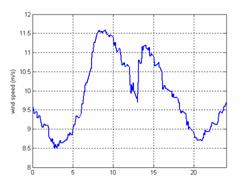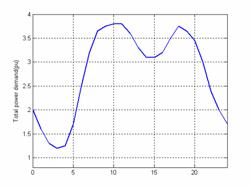Figure 6. Forecast of wind speed Figure 7. Forecast of total power demand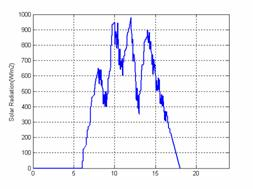Figure 8.Forecast of solar radiation

After the training phase of the neurons network, the simulation results are presented in the figures 9, 10, 11 and 12.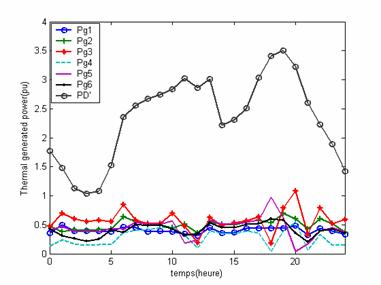Figure 9. Power of the thermal generated nodes and PD’

According to figure 9, we notice that the bus thermal generators powers remain variable within their limits.

Bus generator 2 has a remarkable participate by its active power Pg2 when total power demands P’Dis significant because it is the machine which has the more high cost.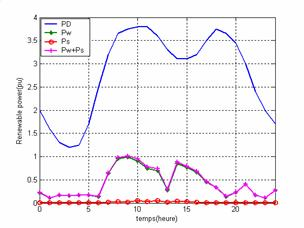Figure 10.Total power demand and renewable power

Figure 10; show the variation of solar thermal power, wind power and their resultant which remains lower than 30%of the total power demand PD.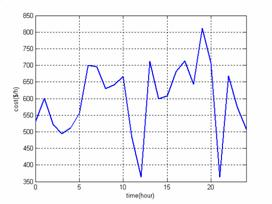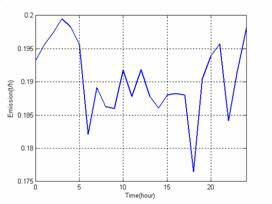Figure 11. Total cost function Figure 12. Emissions function

Figures 11 and 12 who represent respectively the variations of the total cost function and emissions of polluting gases function show that if the emissions of polluting gases decrease in the course of time then the total cost increases and conversely.

Conclusion

In this study we presented a method allowing the resolution of the problem of the Environmental Economic Dispatching of an electrical network including renewable energy sources. We made an optimization without auxiliary elements and the problem consists to extract the maximum of power from the renewable sources and to distribute the remainder of the power on the power stations. To have the solutions of the problem in real time we established them on a neurons network.

References

1.      Granelli GP., Montagna M., Pasini GL., Marannino P., Emission constrained dynamic dispatch. Electr. Power syst. Res., 1992, PP. 56-64, 1992.

2.      Farag A., Al-Baiyat S. and Cheng TC., 1995, Economic load dispatch multi objectives optimization procedures using linear programming techniques. IEEE Trans. On Power Syst., Vol. 10, No.2, PP. 731-738, 1995.

3.      Abido M. A. and Bakhashwain J. M., 2005, Optimal VAR dispatch using a multiobjective evolutionary algorithm. Electrical power and energy systems, PP. 13-20, 2005.

4.      Lin, C.E. and Viviani, G.L.. Hierarchical economic dispatch for piecewise quadratic cost functions, IEEE Transactions on Power Apparatus and Systems, Vol. 103, No. 6, pp. 1170-1175, 1984.

5.      Abido M. A., 2003, A niched Pareto genetic algorithm for multi objectives environmental/economic dispatch. Electrical power and energy systems, PP. 97-105, 2003

6.Piwko, R., Osbom, D., Gramlich, R., Jordan, G., Hawkins, D., and Porter, K. (2005). Wind energy delivery issues, IEEE Power & Energy Magazine, November/December, pp. 67-56, 2005.

7.      Lingfeng Wang and Chanan Singh., 2006, Tadeoff between Risk and Cost in Economic Dispatch Including Wind Power Penetration Using Praticle Swarm Optimisation. International Conference on Power System Technology, 2006.

8.      Miranda, V. and Hang, P. S. (2005). Economic dispatch model with fuzzy constraints and attitudes of dispatchers, IEEE Transactions on Power Systems, Vol. 20, No. 4, Nov., pp. 2143-2145, 2005.

9.      Wang, L. F. and Singh, C. Multi-objective stochastic power dispatch through a modified particle swarm optimization algorithm, Special Session on Applications of Swarm Intelligence to Power Systems, Proceedings of IEEE Swarm Intelligence Symposium, Indianapolis, May, pp. 127-135, 2006.

10.  Zhao et al./ JZhejiang Univ SCI 2005. Multiple objective particle swarm optimisation technique for economic load dispatch’ 6A(5) :420-427.

11.  Hota, P. K. and Dash, S. K. (2004). Multiobjective generation dispatch through a neuro-fuzzy technique, Electric Power Components and Systems, 32: 1191-1206, 2004.

12.  DeMeo, E. A., Grant, W., Milligan, M. R., and Schuerger, M. J. (2005). Wind plant integration: costs, status, and issues, IEEE Power & Energy Magazine, November/December, pp. 38-46, 2004.

13.  Talaq J., El-Hawary F. and El-Hawary M., 1994, A summary of Environmental/Economic Dispatch Algorithms. Trans. On Power Systems, Vol. 6, No. 3, Aug 1994, pp. 1508-1516, 1994.

14.  Faisal A. Mohamed, Heikki N. Koivo., (2007), Online Management of MicroGrid with Battery Storage Using Multiobjective Optimization, POWERENG 2007, April 12-14, Setubal, Portugal, 2007

15.  Bhatnagar R. and Rahmen S., Dispatch of direct load control for fuel cost minimisation. IEEE Trans. on PS, Vol-PWRS-1, pp. 96-102,1986

16.  Dhifaoui R., Hadj Abdallah H. et Toumi B., Le calcul du dispatching économique en sécurité par la méthode de continuation paramétrique. Séminaire à l’I.N.H.Boumerdes Algérie, 1987.

17.  GERGAUD O., Modélisation énergétique et optimisation économique d'un système de production éolien et photovoltaïque couplé au réseau et associé à un accumulateur. Thèse de Doctorat de l’École Normale Supérieure de Cachan, 2002

18.  Guesmi T., Hadj Abdallah H., Ben Aribia H. et Toumi A., Optimisation Multiobjectifs du Dispatching Economique / Environnemental par l’Approche NPGA.International Congress Renewable Energies and the Environment (CERE)’2005 Mars 2005, Sousse Tunisie, 2005.

19.  D. B. Das and C. Patvardhan, ‘ New Multi-objective Stochostic Serch Technique for Economic Load Dispatch, ‘IEE Proc.-Gener. Transm. Distrib., Vol. 145,No. 6, pp.747-752, 1998.

20.  Zahavi J., Eisenberg L., Economic–environmental power dispatch. IEEE Trans. Syst., Vol. 5, No. 5, 1985, PP. 485–489, 1985.

21.  Warsono, D. J. King, and C. S. Özveren, Economic load dispatch for a power system with renewable energy using Direct Search Method, 1228-1233, 2007.

22.  F. Li, JD. Pilgrim, C. Dabeedin, A. Chebbo, and RK. Aggarwal, “Genetic Algorithms for Optimal Reactive Power Compensation on the National Grid System”, IEEE Trans.Power Systems, vol. 20, n° 1, pp. 493-500, February 2005.

23.  Lingfeng Wang, Chanan Singh, 2007, Compromise Between Cost and Reliability in Optimum Design of An Autonomous Hybrid Power System Using Mixed-Integer PSO Algorithm, Department of Electrical and Computer Engineering Texas A&M University

24.  T. BOUKTIR, L. SLIMANI and M. BELKACEMI “A Genetic Algorithm for Solving the Optimal Power Flow Problem”, Leonardo Journal of Sciences, Issue 4, January-June p. 44-58, 2004.

25.  Benjamin Baran, Member, IEEE, José Vallejos, Rodrigo Ramos and Ubaldo Fernandez, Member, IEEE. ‘Reactive Power Compensation using a Multi-objective Evolutionary Algorithm’ PPt 2001, IEEE Porto Power Tech Conference, 10th-13th September, Porto, Portugal.

26.  Mohammad Taghi Ameli, Saeid Moslehpour, Mehdi Shamlo, Economical load distribution in power networks that include hybrid solar power plants, Electric Power Systems Research 78 1147–1152, 2008.

Appendix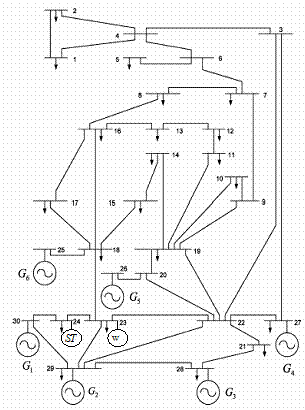Figure 1. Single-line diagram of IEEE 30-bus test system with two renewable power stations

Table 1. Line data

 Line N° Connection Impedance (p.u.) Line N° Connection Impedance (p.u.) 1 30-29 0.0192 + j0.0575 22 13-12 0.0192 + j0.0575 2 30-24 0.0452 + j0.1852 23 12-11 0.0452 + j0.1852 3 29-23 0.0570 + j0.1737 24 19-11 0.0570 + j0.1737 4 24-23 0.0132 + j0.0379 25 19-14 0.0132 + j0.0379 5 29-28 0.0472 + j0.1983 26 19-10 0.0348 + j0.0749 6 29-22 0.0581 + j0.1763 27 19-9 0.0727 + j0.1499 7 23-22 0.0119 + j0.0414 28 10-9 0.0116 + j0.0236 8 28-21 0.0460 + j0.1160 29 16-8 0.1000 + j0.2020 9 22-21 0.0267 + j0.0820 30 9-7 0.1150 + j0.1790 10 22-27 0.0120 + j0.0420 31 8-7 0.1320 + j0.2700 11 22-20 j0.2080 32 7-6 0.1885 + j0.3292 12 22-19 j0.5560 33 6-5 0.2544 + j0.3800 13 20-26 j0.2080 34 6-4 0.1093 + j0.2087 14 23-18 j0.2560 35 3-4 j0.3960 15 18-25 j0.1400 36 4-2 0.2198 + j0.4153 16 18-17 0.1231 + j0.2559 37 4-1 0.3202 + j0.6027 17 18-16 0.0662 + j0.1304 38 2-1 0.2339 + j0.4533 18 18-15 0.0945 + j0.1987 39 27-3 0.0636 + j0.2000 19 17-16 0.2210 + j0.1997 40 22-3 0.0169 + j0.0599 20 15-14 0.0824 + j0.1923 41 20-19 j0.1100 21 16-13 0.1070 + j0.2185

Table 2. Bus data

 Line N° Type Active power (p.u) Reactive power (p.u) Bus voltage (p.u) Line N° Type Active power (p.u) Reactive power (p.u) Bus voltage (p.u) 1 P-Q 0.106 0.019 － 16 P-Q 0.082 0.025 － 2 P-Q 0.024 0.009 － 17 P-Q 0.062 0.016 － 3 P-Q 0.000 0.000 － 18 P-Q 0.112 0.075 － 4 P-Q 0.000 0.023 － 19 P-Q 0.058 0.020 － 5 P-Q 0.035 0.000 － 20 P-Q 0.000 0.000 － 6 P-Q 0.000 0.000 － 21 P-Q 0.228 0.109 － 7 P-Q 0.087 0.067 － 22 P-Q 0.000 0.000 － 8 P-Q 0.032 0.016 － 23 P-Q 0.076 0.000 1.010 9 P-Q 0.000 0.000 － 24 P-Q 0.024 0.000 1.010 10 P-Q 0.175 0.112 － 25 P-V 0.000 0.000 1.071 11 P-Q 0.022 0.007 － 26 P-V 0.000 0.000 1.082 12 P-Q 0.095 0.034 － 27 P-V 0.300 － 1.010 13 P-Q 0.032 0.009 － 28 P-V 0.942 － 1.010 14 P-Q 0.090 0.058 － 29 P-V 0.217 － 1.045 15 P-Q 0.035 0.018 － 30 Bilan 0.000 0.000 1.060